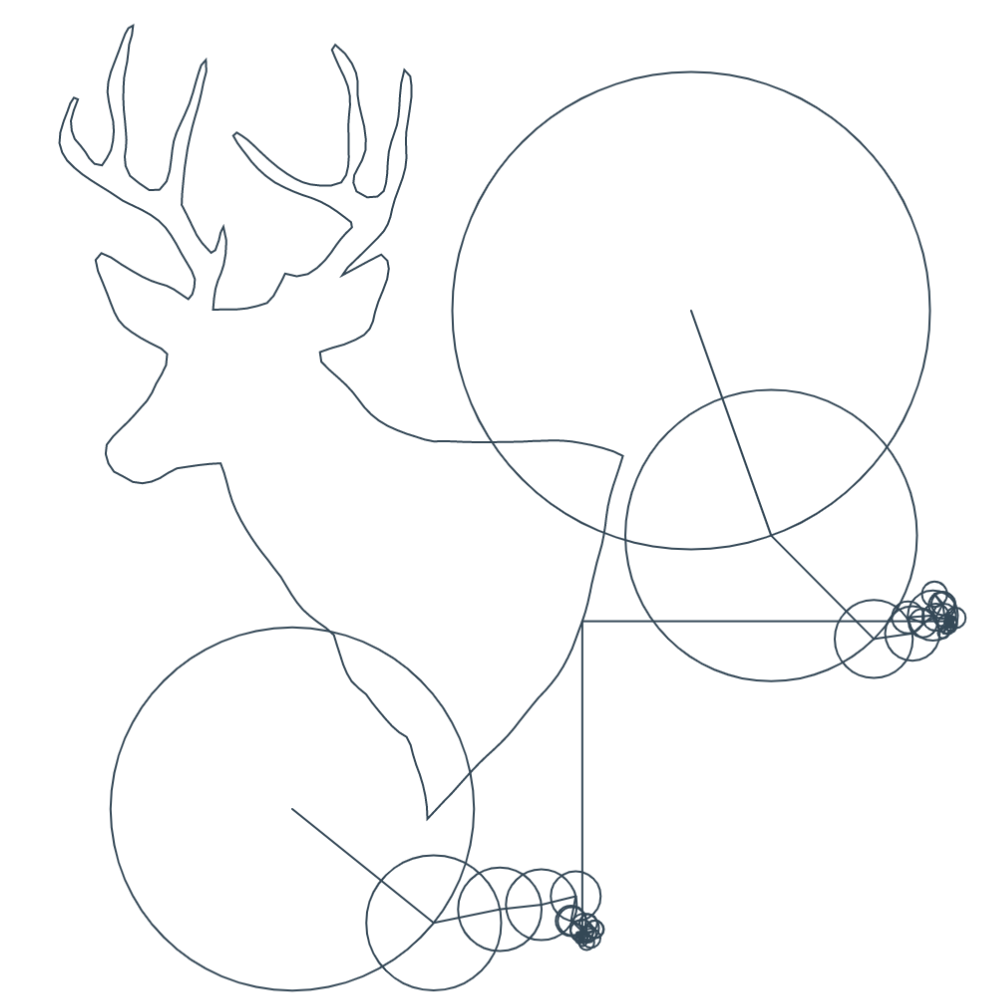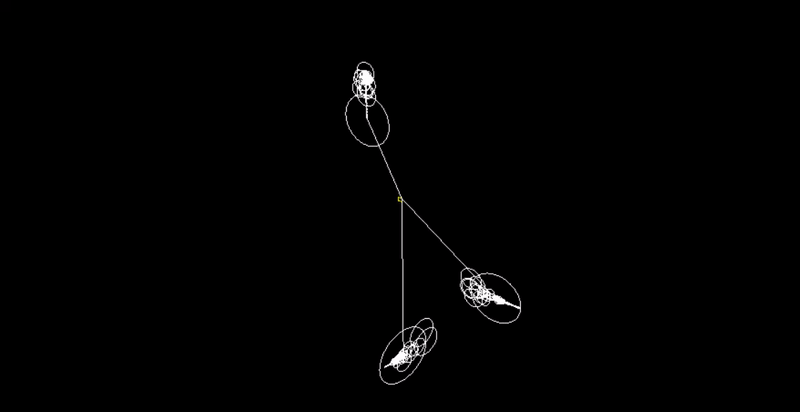# Explanation

## Step 2. Apply the Fourier Transform to each function. This will allow us to represent each function as a sum of revolving circles and vectors. We will use the discrete version of the transform because computers cannot sum up an infinite number of values.## Step 3. Save the output of the Fourier Transform, which is a set of frequencies, amplitudes, and phase shifts. Visually, this is just a circle.## Step 4. Create $3$ epicycle systems from the output above. Each system controls the $x$, $y$, or $z$ coordinate of the points being redrawn.## Step 5. Start an animation that goes from $t = 0$ to $t = 2*pi$. For the $3$ epicycle systems, we sum up $A * cis(f * t + phi)$, where $A$ = amplitude, $f$ = frequency, $t$ = time, $phi$ = phase shift, and $cis(theta) = cos (theta) + i sin(theta)$. We then use the real or imaginary component of the $3$ sums to create a $3D$ point, which is plotted.